Next: *STEP Up: Input deck format Previous: *STATIC   Contents

## *STEADY STATE DYNAMICS

Keyword type: step

This procedure is used to calculate the steady state response of a structure subject to periodic loading. Although the deformation up to the onset of the dynamic calculation can be nonlinear, this procedure is basically linear and assumes that the response can be written as a linear combination of the lowest modes of the structure. To this end, these modes must have been calculated in a previous *FREQUENCY,STORAGE=YES step (not necessarily in the same calculation). In the *STEADY STATE DYNAMICS step the eigenfrequencies, modes, stiffness and mass matrix are recovered from the file jobname.eig.

For harmonic loading the steady state response is calculated for the frequency range specified by the user. The number of data points within this range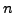can also be defined by the user, default is 20, minimum is 2 (if the user specifiesto be less than 2, the default is taken). If no eigenvalues occur within the specified range, this is the total number of data points taken, i.e. including the lower frequency bound and the upper frequency bound. If one or more eigenvalues fall within the specified range,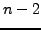points are taken in between the lower frequency bound and the lowest eigenfrequency in the range,between any subsequent eigenfrequencies in the range andpoints in between the highest eigenfrequency in the range and upper frequency bound. Consequently, if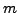eigenfrequencies belong to the specified range,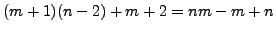data points are taken. They are equally spaced in between the fixed points (lower frequency bound, upper frequency bound and eigenfrequencies) if the user specifies a bias equal to 1. If a different bias is specified, the data points are concentrated about the fixed points. Default for the bias is 3., minimum value allowed is 1. (if the user specifies a value less than 1., the default is taken). The number of eigenmodes used is taken from the previous *FREQUENCY step. Since a steady state dynamics step is a perturbation step, all previous loading is removed. The loading defined within the step is multiplied by the amplitude history for each load as specified by the AMPLITUDE parameter on the loading card, if any. In this context the AMPLITUDE cards are interpreted as load factor versus frequency. Loading histories extending beyond the amplitude frequency scale are extrapolated in a constant way. The absence of the AMPLITUDE parameter on a loading card leads to a frequency independent load.

For nonharmonic loading the loading across one period is not harmonic and has to be specified in the time domain. To this end the user can specify the starting time and the final time of one period and describe the loading within this period with *AMPLITUDE cards. Default is the interval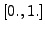and step loading. Notice that for nonharmonic loading the *AMPLITUDE cards describe amplitude versus TIME. Furthermore, the user can specify the number of Fourier terms the nonharmonic loading is expanded in (default:20). The remaining input is the same as for harmonic loading, i.e. the user specifies a frequency range, the number of data points within this range and the bias.

There are two optional parameters: HARMONIC and SOLVER. HARMONIC=YES (default) indicates that the periodic loading is harmonic, HARMONIC=NO specifies nonharmonic periodic loading. The parameter SOLVER determines the package used to solve for the steady state solution in the presence of nonzero displacement boundary conditions. The following solvers can be selected:

• the SGI solver
• PARDISO
• SPOOLES [3,4].
• TAUCS

Default is the first solver which has been installed of the following list: SGI, PARDISO, SPOOLES and TAUCS. If none is installed, an error is issued.

The SGI solver should by now be considered as outdated. SPOOLES is very fast, but has no out-of-core capability: the size of systems you can solve is limited by your RAM memory. With 2GB of RAM you can solve up to 250,000 equations. TAUCS is also good, but my experience is limited to the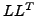decomposition, which only applies to positive definite systems. It has an out-of-core capability and also offers a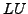decomposition, however, I was not able to run either of them so far. PARDISO is the Intel proprietary solver and is to my knowledge presently the fastest.

First line:

• *STEADY STATE DYNAMICS
• enter any of the parameters you need.
Second line for HARMONIC=YES (default):
• Lower bound of the frequency range (cycles/time)
• Upper bound of the frequency range (cycles/time)
• Number of data points(default: 20)
• Bias (default: 3.)
Second line for HARMONIC=NO:
• Lower bound of the frequency range (cycles/time)
• Upper bound of the frequency range (cycles/time)
• Number of data points(default: 20)
• Bias (default: 3.)
• Number of Fourier terms(default: 20)
• Lower bound of the time range (default: 0.)
• Upper bound of the time range (default: 1.)

```Example:

12000.,14000.,5,4.
```

defines a steady state dynamics procedure in the frequency interval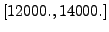with 5 data points and a bias of 4.

```Example: